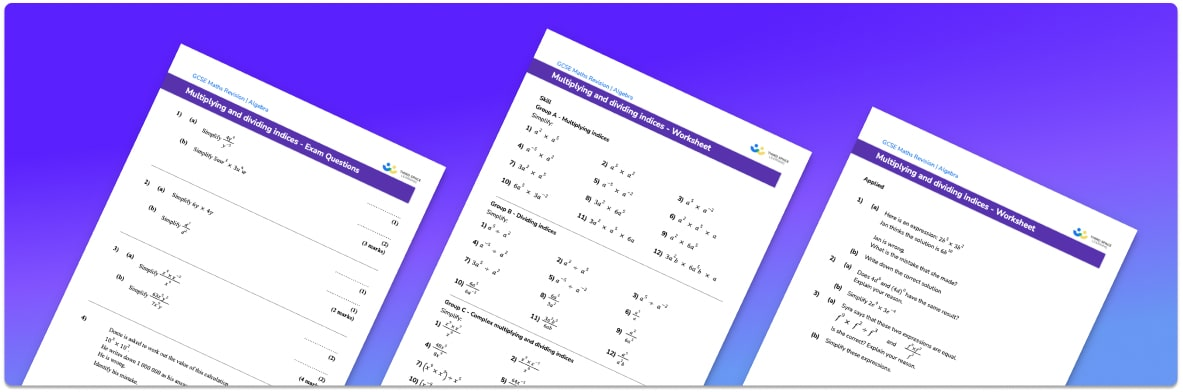# Multiplying Indices Worksheet• Section 1 of the multiplying indices worksheet contains 20+ skills-based multiplying indices questions, in 3 groups to support differentiation
• Section 2 contains 3 applied multiplying indices questions with a mix of worded problems and deeper problem solving questions
• Section 3 contains 3 foundation and higher level GCSE exam style multiplying indices questions
• Answer sheets and a mark scheme for all multiplying indices questions are provided
• Questions follow variation theory with plenty of opportunities for students to work independently at their own level
• All questions created by fully qualified expert secondary maths teachers
• Suitable for GCSE maths revision for AQA, OCR and Edexcel exam boards

• This field is for validation purposes and should be left unchanged.

You can unsubscribe at any time (each email we send will contain an easy way to unsubscribe). To find out more about how we use your data, see our privacy policy.

### Multiplying and dividing indices at a glance

Indices, also called exponents or powers, tell us how many times a number has been multiplied by itself. Indices are written in superscript. We can perform calculations involving indices and we can also simplify expressions involving indices.

When working on calculations involving indices, we must ensure we apply BIDMAS. The I in BIDMAS represents indices so any indices need to be worked out before any multiplication, division, addition or subtraction.

If we have an expression involving multiple terms with the same base number, we can simplify the expression by applying the rules of indices. Two of these rules state that xaxb=xa+b and xaxb=xa-b. When multiplying terms we add the powers and when dividing terms we subtract the powers. These rules also apply to algebraic expressions where unknowns are raised to powers. The rules of indices can be used with powers that are integers, fractions, decimals and with negative powers.

If presented with an expression using index notation but with different base numbers, it may be possible to change the base numbers so that they are the same and which would then allow the expression to be simplified.

Remember that for fractional powers, the denominator indicates the root of the number, which is then raised to the power of the numerator. For example the power of ½ means find the square root and the power of ⅔  means calculate the cube root and then square the answer.

Looking forward, students can progress with more indices worksheets to additional algebra worksheets, for example a simplifying expressions worksheet or simultaneous equations worksheet.For more teaching and learning support on Algebra our GCSE maths lessons provide step by step support for all GCSE maths concepts.

## Do you have KS4 students who need more focused attention to succeed at GCSE?There will be students in your class who require individual attention to help them succeed in their maths GCSEs. In a class of 30, it’s not always easy to provide.

Help your students feel confident with exam-style questions and the strategies they’ll need to answer them correctly with our dedicated GCSE maths revision programme.

Lessons are selected to provide support where each student needs it most, and specially-trained GCSE maths tutors adapt the pitch and pace of each lesson. This ensures a personalised revision programme that raises grades and boosts confidence.

Find out more